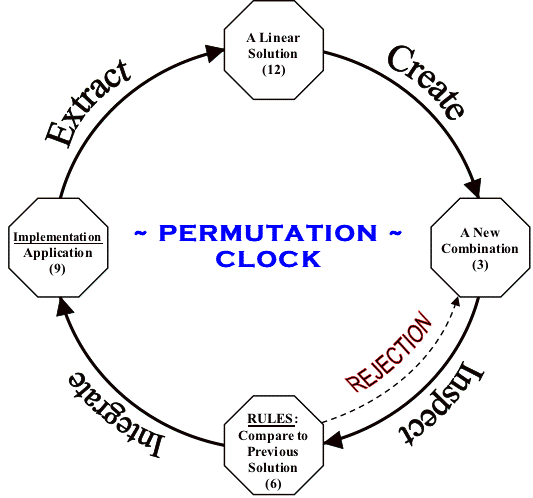# The Countdown Meta-Permutation Class: Presented as Example_05

"Is there a function or class that returns just two index values (i, j) such that SWAP(a[i], a[j]) always produces a unique combination within an array a[]?" ~ Dr. Elaine R. Milito (previously unsolved)

Presented below is the C++ algorithm which answered Dr. Milito's question proposed sometime after the Fall of 1986. Using a target array of size N, two distinct variables (j, i) can be accessed for all head transpositions as well as two distinct variables (q, r) for all tail transpositions. Given two target arrays called h[N], t[N] and the Meta-permutation object s(N), SWAP(h[s.j], h[s.i]) is valid for all head combinations whereas SWAP(t[s.q], t[s.r]) is valid for all tail combinations provided that all SWAP calls are followed by a single s.next() call to calculate four new index values.

Using a nested while loop in the countdown QuickPerm algorithm clearly lead to the development of a Meta-permutation class (below). In contrast, replacing the nested while loop with a conditional if-else statement as described in the counting QuickPerm algorithm causes the Meta-permutation class to "skip" index calculations due to an obvious dependency on the outer loop. Although this conditional substitution can be made regardless of the counting process utilized, the Meta-Permutation Class precludes such a substitution in order to return valid indices.

Example_05 efficiently combines the four previous examples by utilizing a single Meta-Permutation Class aptly named MetaPerm. Here's the Meta-Permutation Class written in C++ for your review:

MetaPerm Class - Combining the Four Previous Examples
 `````` // NOTICE: Copyright 1991-2008, Phillip Paul Fuchs #define N 12 // number of elements to permute. Let N > 2 class MetaPerm { private: unsigned int *p, ax; // index control pointer and index ceiling indicator public: unsigned int i, j, q, r; // declare indexes for head and tail permutations void next(void); // calculate new indexes for head and tail permutations MetaPerm(unsigned int n); // initialize first set of indices (i, j, q, & r) ~MetaPerm(void) { delete [] p; }; }; MetaPerm::MetaPerm(unsigned int n) { p = new unsigned int[n+1]; // declare primary index controller array for(i = 0; i <= n; i++) // initialize primary index controller array p[i] = i; i = 1; // initialize head indices i & j j = 0; ax = n - 1; // define index ceiling q = ax - i; // initialize tail indices q & r r = ax - j; p = 0; // Record initialization event of indices above } // MetaPerm(unsigned int) Constructor (Initialize or Reset Permutation) void MetaPerm::next(void) { // Control Threading Here i = 1; // reset index i to 1 (assumed) while (!p[i]) // while (p[i] == 0) { p[i] = i; // reset p[i] zero value i++; // set new index value for i (increase by one) } p[i]--; // decrease index "weight" for i by one j = i % 2 * p[i]; // IF i is odd then j = p[i] otherwise j = 0 q = ax - i; // adjust tail indices q and r such that q < r r = ax - j; } // MetaPerm::next() /*******************************************************************************************/ /*******************************************************************************************/ void example_05(void) // Combines the four previous examples using one MetaPerm class. { MetaPerm s(N); unsigned int ex1[N], ex2[N], ex3[N], ex4[N]; register unsigned int tmp; for(unsigned int i = 0; i < N; i++) // initialize arrays for permutations { ex1[i] = i + 1; ex2[i] = ex1[i]; ex3[i] = ex1[i]; ex4[i] = N - i; } //display(ex1); // remove comment to display head permutations //display(ex2); // remove comment to display tail permutations //display(ex3); // remove comment to display permutations using head reversals //display(ex4); // remove comment to display permutations using tail reversals while (s.i < N) { tmp = ex1[s.j]; // swap(ex1[s.i], ex1[s.j]) ex1[s.j] = ex1[s.i]; ex1[s.i] = tmp; //display(ex1); // remove comment to display head permutations tmp = ex2[s.r]; // swap(ex2[s.q], ex2[s.r]) ex2[s.r] = ex2[s.q]; ex2[s.q] = tmp; //display(ex2); // remove comment to display tail permutations s.j = 0; // establish lower range for head reversals s.r = N - 1; // establish upper range for tail reversals do // demonstration of permutations using reversals { tmp = ex3[s.j]; // reversal of array head from s.j to s.i ex3[s.j] = ex3[s.i]; ex3[s.i] = tmp; s.j++; s.i--; tmp = ex4[s.r]; // reversal of array tail from s.q to s.r ex4[s.r] = ex4[s.q]; ex4[s.q] = tmp; s.r--; s.q++; } while (s.j < s.i); // or while(s.q < s.r); //display(ex3); // remove comment to display permutations using head reversals //display(ex4); // remove comment to display permutations using tail reversals s.next(); // create new index values for another unique exchange } // while (s.i < N) } // example_05() ``````

MetaPerm Function to Display Permutations (Optional)
 `````` // NOTICE: Copyright 1991-2008, Phillip Paul Fuchs                                           void display(unsigned int *a) { for(unsigned int x = 0; x < N; x++) printf("%d ",a[x]); printf("\n"); // getch(); // Remove comment for "Press any key to continue" } // display() ``````[]

[] | [] | [] | [] | [MetaPerm]
[] | []
[] | [] | []
[]